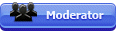## Featured Articles

Check out the latest featured articles.## File Library# Short Cut Formulas For Gas Densities At Various Std.conditions

This topic has been archived. This means that you cannot reply to this topic.
No replies to this topic
|

### #1ankur2061

ankur2061

Gold Member

•• Forum Moderator
•• 2,484 posts

Posted 24 August 2010 - 03:38 AM

Dear all,

I have been posed by many young entry level engineers about gas density calculations which I do in a jiffy using a calculator. I will try to summarize these below:

As a basic we are all supposed to know that gas density is calculated as:

rho = P*M / R*T*Z ------------- 1

or

rho = M / ((R*T*Z) / P) ----------- 2

where:

rho = gas density
M = Molecular weight of gas
R = Universal gas constant
T = Absolute Temperature
P = Absolute Pressure

For different standard conditions the denominator in equation 2, i.e. (R*T*Z/P) varies and below are a few examples:

Standard Conditions 1:

P = 1.01325 bar (abs), T = 15.6 deg C = 288.75 K, Z =1
R = 0.0831447 bara.m3/kmole.K
rho = M / 23.7 kg/m3

Standard Conditions 2:

P = 1.01325 bar (abs), T = 0 deg C = 273.15 K, Z =1
R = 0.0831447 bara.m3/kmole.K
rho = M / 22.414 kg/m3

Note: These are also called normal conditions and the volume defined as Nm3. The term normal is archaic in today's definition of standard conditions of temperature and pressure.

Standard Conditions 3:

P = 1.01325 bar (abs), T = 15 deg C = 288.15 K, Z =1
R = 0.0831447 bara.m3/kmole.K
rho = M / 23.64 kg/m3

Standard Conditions 4:

P = 14.696 psia, T = 60 deg F = 519.67 R, Z =1
R = 10.7316 psia.ft3/lbmole.R
rho = M / 379.5 lb/ft3

Note: The compressibility factor Z is practically unity at all standard conditions mentioned above.

The utility of the standard density is to convert standard volume flow in Sm3 or Scf to mass flow by multiplying it with the standard density, given the standard conditions. It is always good engineering practice to specify the standard conditions in the "Design Basis" document of the particular project being executed. For more details on standard conditions of temperature and pressure please refer the following link:

http://en.wikipedia....re_and_pressure

Regards,
Ankur.

## Recent blog entries on this topic### Short Cut Formulas For Gas Densities At Various Standard Conditions

By ankur2061 in Ankur's_Chemical_Engg_Blog, on 10 February 2012 - 03:42 AM

Dear all,

I have been posed by many young entry level engineers about standard gas density calculations which I do in a jiffy using a calculator. I will try to summarize these below:

As a basic we are all supposed to know that gas density is calculated as:

rho = P*M / R*T*Z ------------- 1

or

rho = M / ((R*T*Z) / P) ----------- 2

where:

rho = gas...